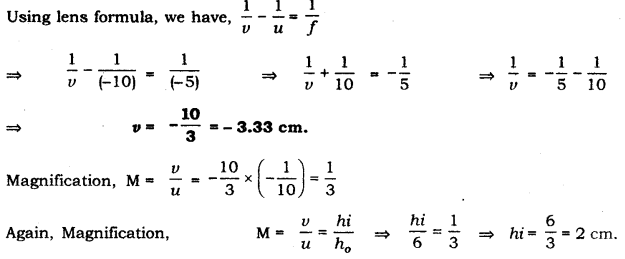# An object of height 6 cm is placed perpendicular to the principal axis of a concave lens of focal length 5 cm

An object of height 6 cm is placed perpendicular to the principal axis of a concave lens of focal length 5 cm. Use lens formula to determine the position, size and nature of the image if the distance of the object from the lens is 10 cm.

We have,|height of object (h) = 6 cm,
focal length of lens (f) = -5 cm and,
object distance (it) = -10 cmThus, the image will be formed in front of the lens at a distance of 3.33 cm from the lens, virtual and erect of size 2 cm.